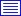# Partners

 A. Schoene

Recent publications
1.  Schoene A., Ziabicki A., Jarecki L., Transient uniaxial orientation of flexible polymer chains in a wide range of elongation rates, POLYMER, ISSN: 0032-3861, DOI: 10.1016/j.polymer.2005.02.109, Vol.46, pp.3927-3935, 2005Abstract:
Affine evolution of chain end-to-end vectors distribution function is derived analytically for non-linear polymer liquids subjected to uniaxial elongational flow, controlled by time-evolution of chain deformation coefficients. Peterlin approximation for non-Gaussian chain elasticity is applied, with Pade` approximation for the inverse Langevin function. The approach enables calculations of transient molecular deformation coefficients in entire range of elongation rates and times. Equations controlling time evolution of the molecular deformation coefficients in elongational flow are solved analytically with an assumption of dominating elongational component. The approach allows to decouple evolution equations and obtain an approximate closed form analytical formula describing time evolution of the molecular deformation with high accuracy, in particular at higher elongation rates, above the Gaussian limit. Predictions of the analytical formula are compared with numerical computations to evaluate the approximation and ranges of its validity. The analytical formula enables predicting evolution of average functions in non-linear systems, such as free energy, tensile stress, molecular orientation, etc. The formula is used to discuss molecular vs. macroscopic deformation in wide range of elongation rates and times, as well as evolution of stress, axial orientation factor, apparent elongational viscosity.

Keywords:
transient molecular deformation, uniaxial elongational flow, non-linear stress-orientation formula

Affiliations:
 Schoene A. - other affiliation Ziabicki A. - IPPT PAN Jarecki L. - IPPT PAN2.  Ziabicki A., Jarecki L., Schoene A., Transient biaxial orientation of flexible polymer chains in a wide range of deformation conditions, POLYMER, ISSN: 0032-3861, DOI: 10.1016/j.polymer.2004.05.070, Vol.45, pp.5735-5742, 2004Abstract:
Transient distribution function of chain ends in non-linear polymer fluids subjected to a constant biaxial flow deformation is approximated by an affine evolution of initial Gaussian distribution function. A non-linear elastic dumbbell potential is used in the evolution equation for the distribution function, with the Peterlin and Pade` approximations of inverse Langevin function. With the approximations, the evolution equation reduces to a system of ordinary first order differential equations for axial components of affine molecular deformation tensor. Numerical and a self-consistent analytical method of solving the system of evolution equations are proposed. Example computations are performed for uniaxial, incompressible elongational flow. The non-linear model covers entire range of deformation rates, and predicts molecular deformation tangential to macroscopic deformation at the beginning of the process, and asymptotically converging to the equilibrium chain deformation in the limit of infinite time. The model describes time evolution of the chain distribution function between the macroscopic affine limit at the beginning of the process and the equilibrium asymptote. The evolution deviates from the asymptotes the more, the lower is the deformation rate. For slow processes, linear Gaussian model is valid, and for very fast ones, solid-like behavior takes place with minor deviation between the molecular and macroscopic deformations, up to the level of full chain extension.

Keywords:
biaxial deformation, transient chain distribution, non-linear molecular orientation

Affiliations:
 Ziabicki A. - IPPT PAN Jarecki L. - IPPT PAN Schoene A. - other affiliation### IPPT PANPawińskiego 5B, 02-106 Warsaw +48 22 826 12 81 (central) +48 22 826 98 15

### Information

© Institute of Fundamental Technological Research Polish Academy of Sciences 2021